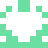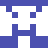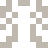### Real Vim ninjas count every keystroke - do you?

###### Pick a challenge, fire up Vim, and show us what you got.

```Your VimGolf key: please sign in

\$ gem install vimgolf
\$ vimgolf setup
\$ vimgolf put 9v0060a458ec000000000205
```

### Make list of numbers from 1 to 30 more compact, but more confusing

Change the naming logic of numbers to end up with less lines.

##### Start file
```one = 1
two = 2
three = 3
four = 4
five = 5
six = 6
seven = 7
eight = 8
nine = 9
ten = 10
eleven = 11
twelve = 12
thirteen = 13
fourteen = 14
fifteen = 15
sixteen = 16
seventeen = 17
eighteen = 18
nineteen = 19
twenty = 20
twenty_one = 21
twenty_two = 22
twenty_three = 23
twenty_four = 24
twenty_five = 25
twenty_six = 26
twenty_seven = 27
twenty_eight = 28
twenty_nine = 29
thirty = 30
```
##### End file
```one = 1
two = 2
three = 3
four = 4
five = 5
six = 6
seven = 7
eight = 8
nine = 9
ten = 10
eleven = [11, 12, 13]
tweleven = [14, 15, 16]
threleven = [17, 18, 19]
twenty = 20
twelve = [21, 22, 23]
twolve = [24, 25, 26]
threlve = [27, 28, 29]
thirty = 30
```

#### View Diff

```11,19c11,13
< eleven = 11
< twelve = 12
< thirteen = 13
< fourteen = 14
< fifteen = 15
< sixteen = 16
< seventeen = 17
< eighteen = 18
< nineteen = 19
---
> eleven = [11, 12, 13]
> tweleven = [14, 15, 16]
> threleven = [17, 18, 19]
21,29c15,17
< twenty_one = 21
< twenty_two = 22
< twenty_three = 23
< twenty_four = 24
< twenty_five = 25
< twenty_six = 26
< twenty_seven = 27
< twenty_eight = 28
< twenty_nine = 29
---
> twelve = [21, 22, 23]
> twolve = [24, 25, 26]
> threlve = [27, 28, 29]
```

### Solutions

The best way to learn is to practice. Below, you will find some of the solutions other golfers have entered. To unlock higher ranked solutions, submit your own entry which does as well or better than the solutions you can currently see - climb the ladder!

Check out these helpful resources to improve your Vim skills... Game on.

Unlock 32 remaining solutions by signing in and submitting your own entry
###### #33 qixiao / @qixiao986 - Score: 254 - 05/30/21 @ 23:09
```11Gllllllllli[jkl<BS><BS><BS><Esc>lla, 12, 14<BS>3]<Esc>jhi[jk<BS><BS><Esc>llla<BS>4, 15, 16]<Esc>jkhhhhhhhhhhhhhhhhiejk<BS><BS><Esc>lllinj<BS><Esc>jd5<CR>ddkothreleven = [17, 19<BS>8, 19]j<BS><Esc>jjkjhhhhi<BS><BS><BS><BS>jk<Esc>kjhhhhhhhdGotwele<BS>ve = [21, 22, 23]<CR>twolve = [27<BS>4, 24, <BS><BS><BS>5, 26]<CR>threlve = [27, 28, 29]<CR>thirty = 30<Esc>:w<CR>:q<CR>
```

## 33 active golfers, 81 entries208
###### #31 - Siddharth Murali / @SidTweetsALess

07/03/2022 at 05:49PM235
###### #32 - oleg / @oleg12573505

01/29/2022 at 03:53PM254
###### #33 - qixiao / @qixiao986

05/30/2021 at 11:09PM